# Accounting for PSF Effects in 2D Image FittingSherpa Threads (CIAO 4.11 Sherpa v1)

## Overview

#### Synopsis:

In this thread, we fit 2-D image data using a Point-Spread-Function (PSF) image as the convolution kernel.

Last Update: 1 Jun 2018 - reviewed for CIAO 4.10, no content change.

## Reading & Filtering Image Data

In this thread, we fit 2-D image data from the FITS datafile center_box_0.25pix.fits. This is the image created from an event file by binning over a region to 0.25 ACIS pixel size with dmcopy; for example:

```unix% dmcopy "event.fits[sky=region(center_box.reg)][bin x=::0.25,y=::0.25]" \
center_box_0.25pix.fits
```

This dataset is input into Sherpa with the load_data command:

```sherpa> load_data("center_box_0.25pix.fits")
```

Now the dataset may be displayed:

```sherpa> image_data()
```

The input data image is shown in Figure 1.

### Figure 1: Input data image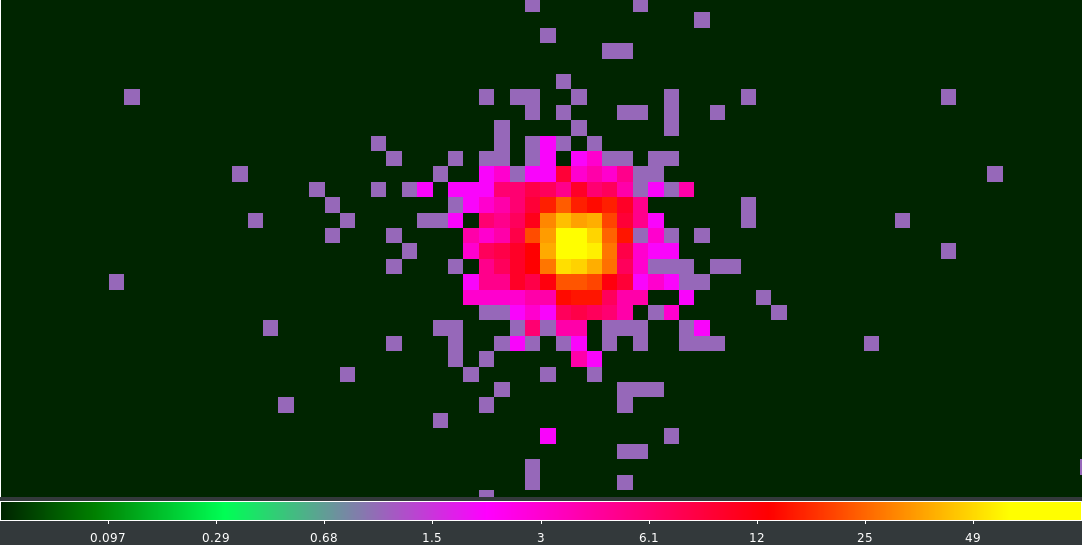### Figure 1: Input data image

Image data may be filtered in Sherpa interactively with DS9. After the data have been displayed, go to the Region menu in DS9 and choose a designated region shape. In this example, we use the box shape. When the box is displayed, the size of the box can be changed with the cursor or within the information window which is opened via the Region→Get Information menu item, as shown in Figure 2.

### Figure 2: Selecting box region in DS9### Figure 2: Selecting box region in DS9

After the desired region size is set, the region can be used to filter the data as follows:

```sherpa> image_getregion()
'box(88.16875,75.8625,70.416667,68.508334,0);'
sherpa> notice2d("rotbox(88.16875,75.8625,70.416667,68.508334,0)")
```Python 3 bytes strings

In Python 3, Sherpa may return bytes strings instead of regular unicode strings, which is denoted with a 'b' prior to the string.

```sherpa> image_getregion()
b'box(88.16875,75.8625,70.416667,68.508334,0);'
```

If a bytes string is returned, use the Python decode operation to convert the string to unicode.

```sherpa> image_getregion().decode()
'box(88.16875,75.8625,70.416667,68.508334,0);'
```

In this case, the dataset is using image coordinates, so we have to make sure that DS9 is set to interact with Sherpa in physical coordinates by setting AnalysisCIAOCoordsImage.

```sherpa> show_all()
Data Set: 1
Filter: Box(88.1688,75.8625,70.4167,68.5083)
name      = center_box_0.25pix.fits
x0        = Float64
x1        = Float64
y         = Float64
shape     = (147, 169)
staterror = None
syserror  = None
sky       = physical
crval    = [ 4075.    4039.25]
crpix    = [ 0.5  0.5]
cdelt    = [ 0.25  0.25]
eqpos     = world
crval    = [ 248.6211   70.531 ]
crpix    = [ 4096.5  4096.5]
cdelt    = [-0.0001  0.0001]
crota    = 0
epoch    = 2000
equinox  = 2000
coord     = logical
```

The same filter can be set on the command line with the region definition if it is already known. Note that Sherpa requires the Image coordinates (and not Physical coordinates) to be used in region definitions:

```sherpa> notice2d("box(88.16875,75.8625,70.416667,68.508334)")
```

## Defining the Instrument Model with a PSF Image

An instrument model, named psf0, may be established using an image of a PSF. The PSF image is loaded with the load_psf command, then added to the list of models in the session as a PSF instrument model with set_psf.

```sherpa> load_psf("psf0","psf_f1_norm_0.25pix.fits")

sherpa> set_psf("psf0")

sherpa> show_psf()
PSF Model: 1
name      = psf_f1_norm_0.25pix.fits
x0        = Float64
x1        = Float64
y         = Float64
shape     = (256, 256)
staterror = None
syserror  = None
sky       = physical
crval    = [ 4064.5  4026.5]
crpix    = [ 0.5  0.5]
cdelt    = [ 0.25  0.25]
eqpos     = world
crval    = [ 248.6211   70.531 ]
crpix    = [ 4096.5  4096.5]
cdelt    = [-0.0001  0.0001]
crota    = 0
epoch    = 2000
equinox  = 2000
coord     = logical

sherpa> print(psf0)
psfmodel.psf0
Param        Type          Value          Min          Max      Units
-----        ----          -----          ---          ---      -----
psf0.kernel  frozen psf_f1_norm_0.25pix.fits
psf0.size    frozen   (169, 147)   (169, 147)   (169, 147)
psf0.center  frozen   (128, 128)   (128, 128)   (128, 128)
psf0.norm    frozen            1            0            1
```

Note that the PSF is automatically renormalized to 1. Renormalization is done by summing over all image pixels, regardless of the setting of xsize and ysize. To learn more about 2-D PSF instrument models, please read the ahelp file by typing:

```sherpa> ahelp "set_psf"
```

The PSF image file for this example was created using the CIAO tool mkpsf, which is no longer available as of the CIAO 4.5 release in Decemeber 2012. The best PSF image can be created with The Chandra Ray Tracer (ChaRT). The file center_box_0.25pix.fits was used as input to mkpsf in order to match the binning of the resulting PSF image file (psf_f1_norm_0.25pix.fits). Sherpa requires that the binning of the PSF image file match the binning of the input data image.

To view the PSF image, load it into DS9 with the Sherpa image_psf command.

```sherpa> image_psf()
```

The PSF image is shown in Figure 3.

### Figure 3: PSF image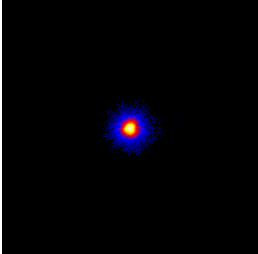### 2-D PSF instrument model terms and parameters

PSF: The point spread function (PSF) is defined by the full (unfiltered) PSF image loaded into Sherpa (or the PSF model expression evaluated over the full range of the dataset; both types of PSFs are established with the load_psf command). The PSF may be displayed with the show_psf command, and visualized with image_psf, plot_psf, and contour_psf.

kernel: The kernel is the sub-section of the PSF image (or model) which is used to convolve the data. This sub-section is created from the PSF when the size and center of the kernel are defined by set_psf. The PSF kernel information may be displayed with the show_kernel command, and visualized with image_kernel, plot_kernel, and contour_kernel.

• size:

The PSF image provided may be much larger than the instrument PSF size (in number of pixels), and therefore larger than needed. In order to speed the convolution process—by restricting the number of points within the PSF that Sherpa must evaluate—a square, sub-image kernel is specified. In this example, the input PSF image file has 256x256 pixels, but the portion that will be used for the convolution is only the center 32x32 pixels sub-image (note that the x and y size parameters need not be equal; rectangular PSFs are supported).

```sherpa> psf0.size = [32,32]
sherpa> psf0.center = [128,129]
sherpa> set_psf(psf0)
sherpa> show_kernel()
psfmodel.psf0
Param        Type          Value          Min          Max      Units
-----        ----          -----          ---          ---      -----
psf0.kernel  frozen psf_f1_norm_0.25pix.fits
psf0.size    frozen     (32, 32)     (32, 32)     (32, 32)
psf0.center  frozen   (128, 129)   (128, 129)   (128, 129)
psf0.norm    frozen            1            0            1

```

Note that the sub-image will be empty if the PSF centroid is located outside the sub-image.

In some cases, a larger sub-image will be needed, such as when the source is located off-axis, or when including the PSF wings is important for the analysis. We may update the parameters to increase the PSF fraction included in the sub-image:

```sherpa> set_psf(psf0)
sherpa> psf0.size = [72,72]
sherpa> print(get_psf())
psfmodel.psf0
Param        Type          Value          Min          Max      Units
-----        ----          -----          ---          ---      -----
psf0.kernel  frozen psf_f1_norm_0.25pix.fits
psf0.size    frozen     (72, 72)     (72, 72)     (72, 72)
psf0.center  frozen   (128, 129)   (128, 129)   (128, 129)
psf0.norm    frozen            1            0            1

sherpa> image_psf()
```

The instrument PSF sub-images may be displayed with the image_psf command, as shown in Figure 4.

### Figure 4: PSF sub-image for convolution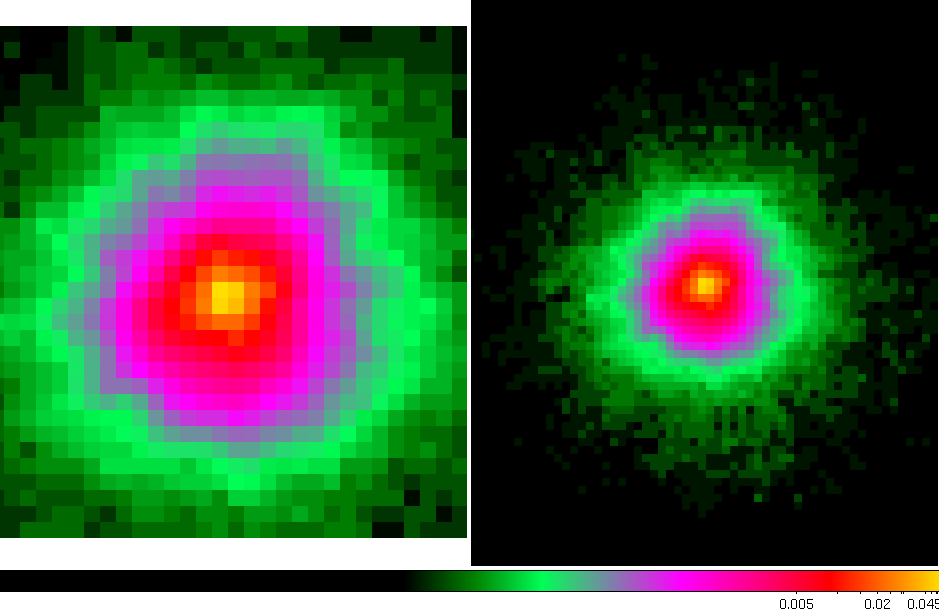### Figure 4: PSF sub-image for convolution

The left panel shows the smaller sub-image of 32x32 pixels, and the right panel shows an expanded to 72x72 pixels sub-image. Notice that the larger sub-image contains a significant amount of the PSF wings structure.

• center:

The kernel centroid must always be at the center of the extracted sub-image. Otherwise, systematic shifts will occur in best-fit positions of point sources, etc. The parameter center=[center0,center1] determines the location of the original PSF image on which the sub-image is centered.

• norm:

The PSF image model array is renormalized to 1 by default, unless the parameter 'norm' is 0; norm=0 produces the functionality of a 2-D kernel model. Renormalization is done by summing over all image pixels, regardless of the x and y size values.

• origin: [optional]

A Sherpa PSF model includes an optional, hidden 'origin' attribute which allows the user to choose which pixel in the PSF kernel to mark as the origin; Sherpa sets the origin to the brightest pixel in the kernel by default. The psf.origin parameter of a PSF model is set using the (x,y) coordinates of a chosen pixel in the PSF kernel, but decremented by one in both x and y directions. For example, if DS9 was used to find that the brightest pixel is at (122, 131), psf.origin should be set equal to '(121, 130)'. This is necessary because Sherpa internally stores data, PSF, and kernel information as NumPy arrays—which start counting from element 0—whereas DS9 uses pixel coordinates, with the first pixel having a value of (1, 1).Caution

In order to appropriately use the psf.origin attribute, the axes of the PSF image must both be even numbers. That is, if the axes of the PSF image are [244, 262] pixels, then there would be no problems setting the psf.origin; but for axes lengths of [243, 262], [244, 261], or [243, 261] pixels, the set psf.origin value gets shifted by one-pixel from the intended origin of the kernel.

When using the Sherpa 2-D PSF model, the user should ensure that the PSF file contains an image where the length of each axis is an even number.

If psf.origin is not set by the user—leaving Sherpa to automatically locate the position of the brightest pixel in the kernel and set this as the origin—Sherpa will find the correct location, provided that a) the brightest pixel should coincide with the origin in the analysis, and b) that there is only one pixel that has that brightest value.

The radial parameter of the PSF model is not applicable to 2-D PSFs; to learn about its use in 1-D PSF models, refer to the set_psf ahelp file.

## Defining a Multi-Component Source Model Expression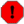Warning

Do not fit 2-D models with world coordinates in Sherpa. 2-D fitting should only be done in image or physical coordinates. After a fit is completed, then the user may convert the fitted position to RA and Dec, and convert the other parameters (e.g. FWHM) to the desired units (e.g. arcsec).

Now we will define a source model expression using a two-dimensional Gaussian function (gauss2d) and a constant (const2d), which will be convolved by the PSF model using Fast Fourier Transform (FFT). We will then define reasonable initial parameter values to begin the fitting (note that the Sherpa guess command may also be used).

```sherpa> set_source(const2d.c1 + gauss2d.g1)
sherpa> c1.c0 = 1.0
sherpa> g1.fwhm = 6
sherpa> g1.xpos = 90
sherpa> g1.ypos = 80
sherpa> g1.ampl = 100

sherpa> show_source()
Model: 1
(const2d.c1 + gauss2d.g1)
Param        Type          Value          Min          Max      Units
-----        ----          -----          ---          ---      -----
c1.c0        thawed            1            0  3.40282e+38
g1.fwhm      thawed            6  1.17549e-38  3.40282e+38
g1.xpos      thawed           90 -3.40282e+38  3.40282e+38
g1.ypos      thawed           80 -3.40282e+38  3.40282e+38
g1.ellip     frozen            0            0        0.999
g1.theta     frozen            0     -6.28319      6.28319    radians
g1.ampl      thawed          100 -3.40282e+38  3.40282e+38
```

The model component c1 is interpreted as the constant background contribution to the data. We can also see how the source model is convolved with the PSF by using the show_model command:

```sherpa> show_model()
PSF Model: 1
name      = psf_f1_norm_0.25pix.fits
x0        = Float64
x1        = Float64
y         = Float64
shape     = (256, 256)
staterror = None
syserror  = None
sky       = physical
crval    = [ 4064.5  4026.5]
crpix    = [ 0.5  0.5]
cdelt    = [ 0.25  0.25]
eqpos     = world
crval    = [ 248.6211   70.531 ]
crpix    = [ 4096.5  4096.5]
cdelt    = [-0.0001  0.0001]
crota    = 0
epoch    = 2000
equinox  = 2000
coord     = logical

Model: 1
(psfmodel.psf0) o ((const2d.c1 + gauss2d.g1))
Param        Type          Value          Min          Max      Units
-----        ----          -----          ---          ---      -----
c1.c0        thawed            1            0  3.40282e+38
g1.fwhm      thawed            6  1.17549e-38  3.40282e+38
g1.xpos      thawed           90 -3.40282e+38  3.40282e+38
g1.ypos      thawed           80 -3.40282e+38  3.40282e+38
g1.ellip     frozen            0            0        0.999
g1.theta     frozen            0     -6.28319      6.28319    radians
g1.ampl      thawed          100 -3.40282e+38  3.40282e+38

```

## Modifying Statistic Setting

Since the data being fit has low counts, we will change the fit statistic to cash:

```sherpa> set_stat("cash")
```

Note that truncation is turned on when the Cash statistic is used; this setting prevents negative model-predicted data values from affecting the convergence process.

Further details about the Cash statistic method are available by typing:

```sherpa> ahelp "cash"
```

## Fitting

First, the data is fit assuming a constant, frozen background component. We also want to change the fit optimization method from the the default method of Levenberg-Marquardt (levmar) to Nelder-Mead Simplex, since the former is not robust with our choice of statistics.

```sherpa> c1.c0 = 1.0
sherpa> freeze(c1.c0)
sherpa> set_method_opt("finalsimplex",0)
sherpa> fit()
Dataset               = 1
Statistic             = cash
Initial fit statistic = 7648.86
Final fit statistic   = 4066.78 at function evaluation 296
Data points           = 4899
Degrees of freedom    = 4895
Change in statistic   = 3582.08
g1.fwhm        2.80105
g1.xpos        88.6612
g1.ypos        77.227
g1.ampl        166.653
```

The fit is run again with the background component thawed:

```sherpa> thaw(c1.c0)
sherpa> fit()
Dataset               = 1
Statistic             = cash
Initial fit statistic = 4066.78
Final fit statistic   = -4457.71 at function evaluation 551
Data points           = 4899
Degrees of freedom    = 4894
Change in statistic   = 8524.49
c1.c0          0.0027774
g1.fwhm        3.73819
g1.xpos        88.5798
g1.ypos        77.2406
g1.ampl        113.113
```

Now, we will get the confidence level of the fit, and in doing so, recalculate the best-fit parameters using the confidence method.

```sherpa> conf()
g1.ypos lower bound:	-0.0679715
g1.xpos lower bound:	-0.068848
g1.ypos upper bound:	0.0679715
g1.xpos upper bound:	0.068848
g1.ampl lower bound:	-8.9519
g1.ampl upper bound:	10.2147
c1.c0 lower bound:	-0.00125664
c1.c0 upper bound:	0.00146963
g1.fwhm lower bound:	-0.151732
g1.fwhm upper bound:	0.151732
Dataset               = 1
Confidence Method     = confidence
Iterative Fit Method  = None
Statistic             = cash
confidence 1-sigma (68.2689%) bounds:
Param            Best-Fit  Lower Bound  Upper Bound
-----            --------  -----------  -----------
c1.c0           0.0027774  -0.00125664   0.00146963
g1.fwhm           3.73819    -0.151732     0.151732
g1.xpos           88.5798    -0.068848     0.068848
g1.ypos           77.2406   -0.0679715    0.0679715
g1.ampl           113.113      -8.9519      10.2147
```Fitting Multiple Data Sets with Differing PSFs

Note that as of the CIAO 4.2 Sherpa version 2 release in July 2010, it is possible to simultaneously fit multiple data sets where each is fit using a different PSF model. In order to do this, the load_psf command would be called for each separate PSF model required for fitting, and then set_psf would be used to assign the appropriate PSF model to each data set.

```sherpa> load_psf("psf1", "gauss_psf.fits")
sherpa> set_psf(psf1)
sherpa> set_source(const2d.c1 + gauss2d.g1)

sherpa> set_psf(2, psf2)
sherpa> set_source(2, const2d.c2 + gauss2d.g2 + beta2d.b2)

sherpa> fit()
```

In the example above, PSF model 'psf1' is convolved with the source model for data set 1, and PSF model 'psf2' is convolved with the source model for data set 2, when 'fit()' is called to simultaneously fit data sets 1 and 2.

## Examining Fit Results

The fit results may be examined with the image_fit command:

```sherpa> image_fit()
```

This command displays the data (upper left), best fit model (upper right), and residuals (lower left) in three DS9 frames, as shown in Figure 5.

### Figure 5: Data, best-fit model, and residuals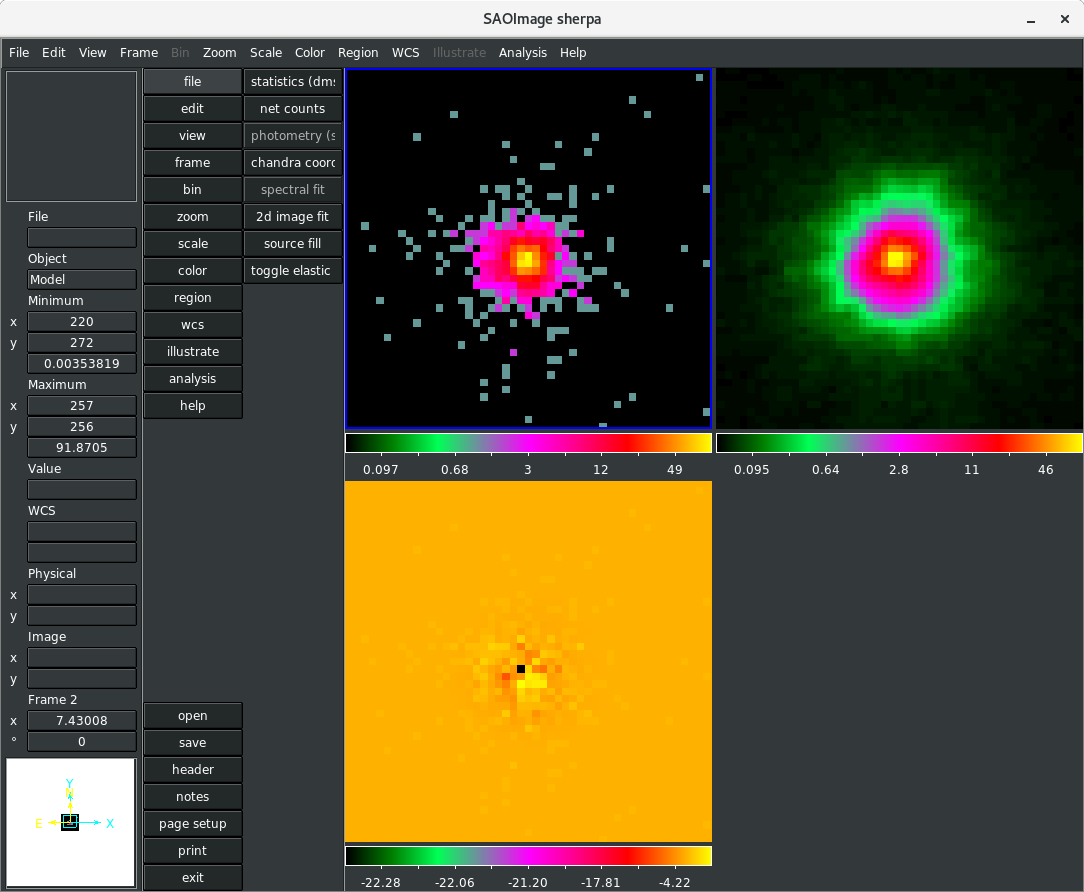### Figure 5: Data, best-fit model, and residuals

This montage of images can be used to see how well the model describes the data.

The residuals may also be examined using a contour plot:

```sherpa> contour_resid()
sherpa> limits(X_AXIS, 55, 120)
sherpa> limits(Y_AXIS, 45, 110)
sherpa> get_contour_zrange()
[-12.236134038141355, 12.765477126550415]
```

The resulting contour plot is shown in Figure 6; get_contour_zrange() provides the minimum and maximum values along the z-axis of the plot.

### Figure 6: Contour plot of the residuals### Figure 6: Contour plot of the residuals

The image model and residual data (in counts) may be written to external FITS image files with the following commands:

```sherpa> save_model("2dpsf_model_cnt.fits")
sherpa> save_resid("2dpsf_resid_cnt.fits")
```

The residuals file "2dpsf_resid_cnts.fits" may be analyzed for any significant difference between the model and the data. It can also be smoothed with aconvolve for better visual inspection.

Note that the effects of 2-D blurring in a 2-D image cannot be reproduced by convolving the radial profile of the PSF with a profile of the model.

## Scripting It

The file fit.py is a Python script which performs the primary commands used above; it can be executed by typing exec(open("fit.py").read()) on the Sherpa command line.

The Sherpa script command may be used to save everything typed on the command line in a Sherpa session:

```sherpa> script(filename="sherpa.log", clobber=False)
```

(Note that restoring a Sherpa session from such a file could be problematic since it may include syntax errors, unwanted fitting trials, et cetera.)

The CXC is committed to helping Sherpa users transition to new syntax as smoothly as possible. If you have existing Sherpa scripts or save files, submit them to us via the CXC Helpdesk and we will provide the CIAO/Sherpa 4.11 syntax to you.